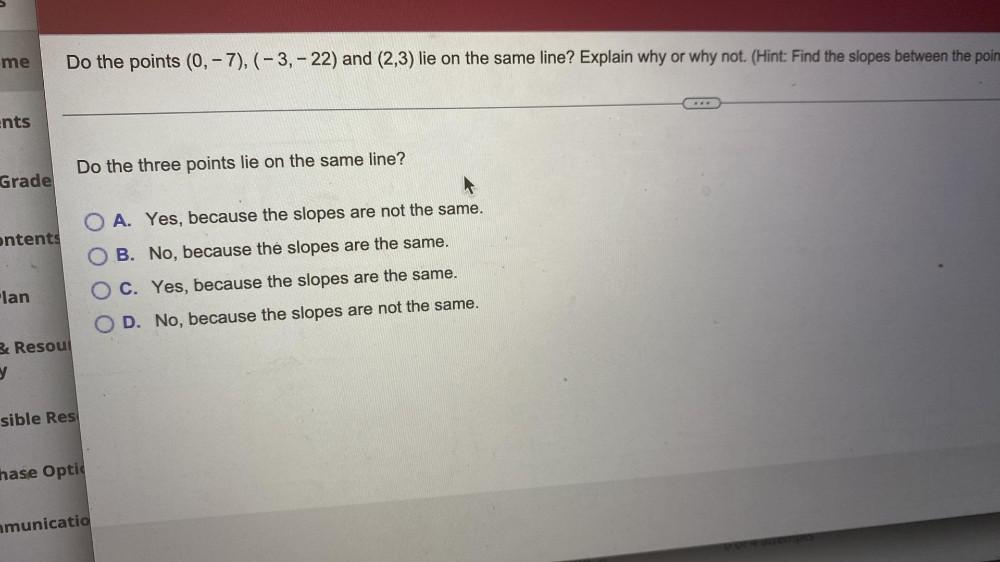Question:

# Do the points (0, -7), (-3,- 22) and (2,3) lie on the same line? Explain why or why not. (Hint: Find the slopes between the points). Do the three points lie on the same line? (A) Yes, because the slopDo the points (0, -7), (-3,- 22) and (2,3) lie on the same line? Explain why or why not. (Hint: Find the slopes between the points). Do the three points lie on the same line? (A) Yes, because the slopes are not the same. (B) No, because the slopes are the same. (C) Yes, because the slopes are the same. (D) No, because the slopes are not the same.